Science, Maths & Technology

### Become an OU studentGeometry

Start this free course now. Just create an account and sign in. Enrol and complete the course for a free statement of participation or digital badge if available.

# 1.4.2 Alternate angles

Other pairs of equal angles can be identified in Example 5. These pairs of angles occur in a Z-shape, as indicated by the solid line in the diagram below. Such angles are called alternate angles.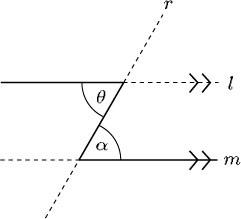When a line intersects two parallel lines, alternate angles are equal.

To prove this result consider the diagram below: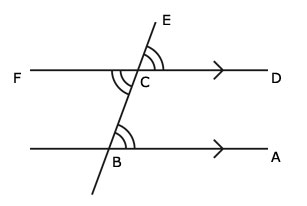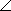ABC =DCE (corresponding angles)

andDCE =FCB (vertically opposed angles)

So,ABC =FCB (both equal toDCE).

The other two angles are also equal and are also called alternate angles.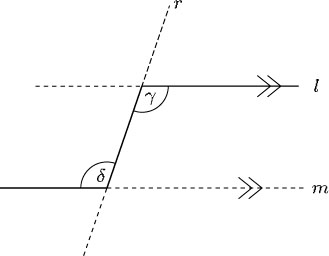It is important to realise that you can find the sizes of unknown angles in many shapes by using a combination of the angle properties that have been outlined. To recap:

• Vertically opposite angles are equal.

• Angles at a point add up to 360°.

• Angles on a straight line add up to 180°.

• Corresponding angles on parallel lines are equal.

• Alternate angles on parallel lines are equal.

## Example 6

Find α and β in the following diagram.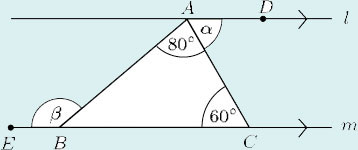Line l is parallel to line m, therefore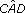and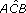are alternate angles. So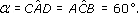Similarly,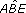and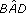are alternate angles. But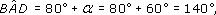and hence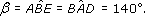These properties of corresponding and alternate angles mean that the opposite angles in a parallelogram are also equal.